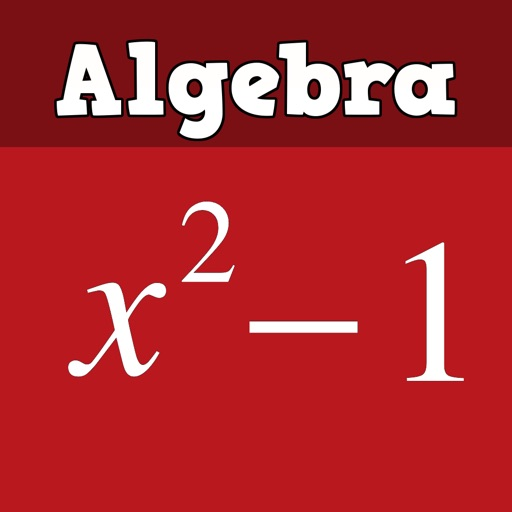Algebra isn an app that teaches you how to do algebra with worked, step-by-step examples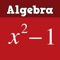Algebra Study Guide LT

by Flamethrower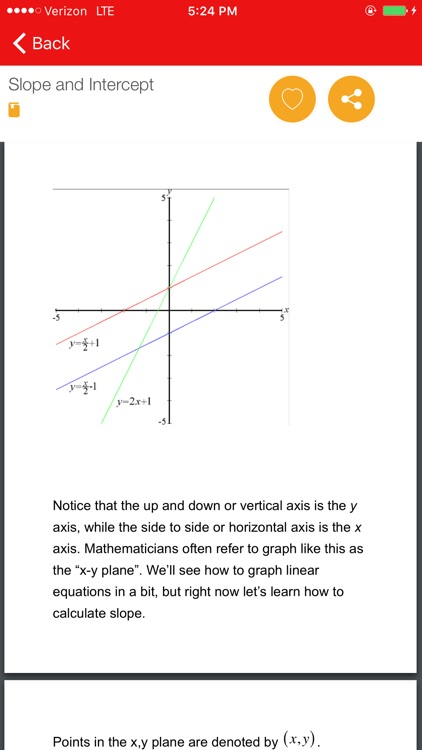Algebra isn an app that teaches you how to do algebra with worked, step-by-step examples. If algebra leaves you confused, Algebra app is here to help with hundreds of worked examples that show step-by-step solutions. This Algebra app is practical - it will help you learn algebra by seeing how an expert solves problems. Includes both books to read at your own pace and video tutorials.App Details

Version
1.1
Rating
(3)
Size
28Mb
Genre
Education Reference
Last updated
June 7, 2017
Release date
September 11, 2016

App Screenshots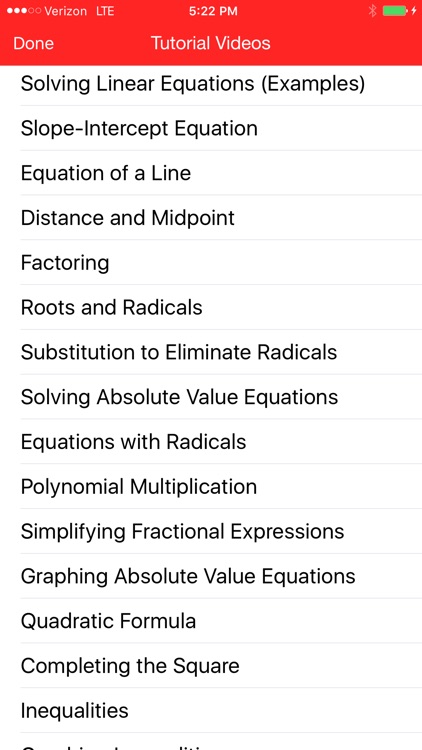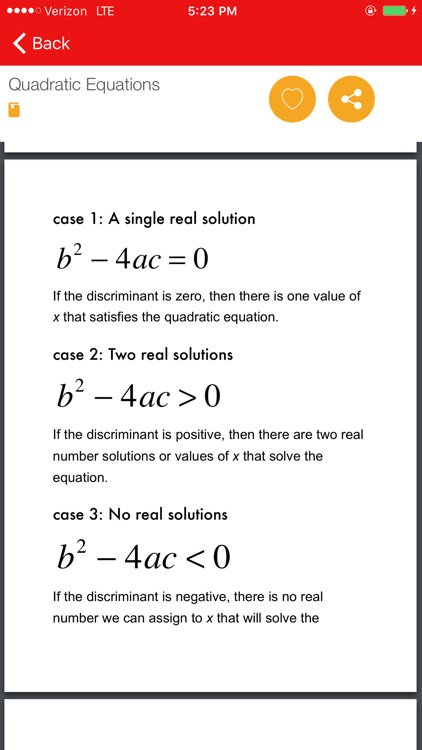App Store Description

Algebra isn an app that teaches you how to do algebra with worked, step-by-step examples. If algebra leaves you confused, Algebra app is here to help with hundreds of worked examples that show step-by-step solutions. This Algebra app is practical - it will help you learn algebra by seeing how an expert solves problems. Includes both books to read at your own pace and video tutorials.

- Review of numbers and exponents
- Solving for variables
- Factoring
- Rational functions
- Simplifying
- Doing word problems
- Completing the Square
- Solving cubics

Video Tutorials Include:
-Exponents and Absolute Values
- Solving for unknown variables
- Adding, Subtracting, Multiplying and Dividing Polynomials
- Slope-Intercept Equation
- Equation of a Line
- Distance and Midpoint
- Solving Absolute value equations, graphing absolute values
- Simplifying Fractional Expressions
- Completing the Square
- Inequalities
- Interest rate and percentage problems
- Word Problems
- Logarithms
- Solving Simultaneous Equations

FEATURES:
- Easy to understand and learn algebra at your own pace
- Good for all levels of algebra, from algebra I to college algebra
- Great for students or for review and self-study
- Randomly selects a different lesson each day you open the app, or view lessons in order